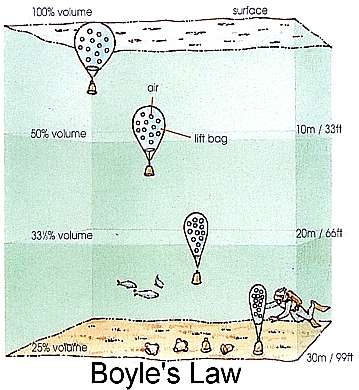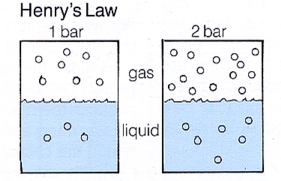DIVE MEDICINE

Before we go into details of dive medicine, we first need to know what effect the presesence of different gas in air has on diving. Hence we need to understand some basic physics of gases starting with the gas laws.• Boyle's law ( P µ 1/V ) states that for a fix mass of gas at constant temperature, the pressure, is inversely proportional to the volume.

• Dalton's law on partial pressure states that in a mixture of gases, each gas exerts a pressure proportional to it's concentration. (Ptotal = P1 + P2 + P3 + ...... )

• Constant volume ( P µ T ) law states that if the volume is fixed, pressure is directly proportional to the temperature.• Charles' law ( V µ T )  states that for a fixed mass of gas at constant pressure, volume is directly proportional to the temperature.• Henry's Law states that at constant temperature, the amount of gas which dissolves in a liquid with which it is in contact is proportional to the partial pressure of the that gas.

Absorption and Release of gases by our body tissues are dependent on the above mentioned laws and can determine whether a diver develops problems such as decompression sickness (DCS), nitrogen narcosis and toxic gas effects. The human body cells are composed mainly of water and small amounts of lipids. At body temperature, both water and lipids behave as liquids, facilitating the absorption of gases into our tissues. Different tissues also have different coefficients of solubility for different gases. The absorption of gases is governed by Henry's Law.

 DCS Nitrogen Narcosis Gas toxicity Barotrauma